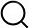# Also in the Article

Experimental setup and data analysis
This protocol is extracted from research article:
On-demand photonic entanglement synthesizer

Procedure

We used a continuous-wave Ti:sapphire laser at 860 nm. Our optical parametric oscillator [OPO; the same design as in (31)] produced a squeezed light with ~5 dB of squeezing and ~8 dB of anti-squeezing at low frequencies (this means ~9 dB of pure squeezing and the total loss of ~20% during the generation and measurement process). Our loop, built by a Herriott-type optical delay line (32), has a round-trip length of 19.8 m and a round-trip loss of ~7% . Considering this loop length, we artificially divided the squeezed light into 66-ns time bins. Each time bin was further divided into 20-ns switching time used for changing system parameters and 46-ns processing time within which a squeezed optical pulse was defined. The loop includes a variable beam splitter composed of two polarizing beam splitters (PBSs) and one bulk-type polarization-rotation EOM. By inserting a quarter wave plate (QWP) between the PBSs, the transmissivity of the beam splitter was initially set to 0.5, and the EOM changed the transmissivity when it was triggered. The variable phase shifter was realized by a bulk-type phase-modulation EOM, shifting the phase from the initially locked value of 0 when it was triggered. Last, the pulses after the loop were mixed with a local oscillator (LO) beam and measured using a homodyne detector. Here, a waveguide-type EOM in the LO’s path can shift the phase ϕ(t) and thereby change the measurement basis $x̂ϕ(t)$. During the measurement, we periodically switched between two different settings at a rate of 2 kHz; one is the feedback setting when the cavity length of the OPO and the relative phases of beams were actively locked by weakly injected reference beams, and the other is the measurement setting when the control sequence in Fig. 2B was triggered and the data were acquired without the reference beams (12).

To analyze the generated states, we acquired the homodyne detector’s signal using an oscilloscope at a sampling rate of 1.25 GHz. Five thousand data frames were recorded to estimate each inseparability parameter and nullifier. The quadrature of the k-th mode was extracted by applying a temporal mode function fk(t) to each data frame, defined as (33)$fk(t)∝{e−γ2(t−tk)2(t−tk)(2∣t−tk∣≤T)0(otherwise)$(2)and normalized to be $∫−∞∞‍∣fk(t)∣2dt=1$. The parameters used in this experiment are T = 46 ns, γ = 6 × 107 /s, and tk = t0 + (k − 1)τ, where t0 is the optimized center position of the first mode and τ = 66 ns is the interval between the modes. Using these parameters, we checked the orthogonality of the neighboring modes by applying fk(t) to the data frames for the shot noise signal and confirming that the quadrature correlation between different modes is negligible (33).

Note: The content above has been extracted from a research article, so it may not display correctly.

Q&A